## Figurate Number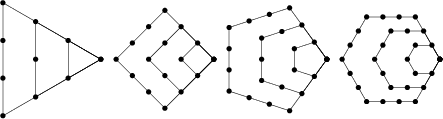A number which can be represented by a regular geometrical arrangement of equally spaced points. If the arrangement forms a Regular Polygon, the number is called a Polygonal Number. The polygonal numbers illustrated above are called triangular, square, pentagonal, and hexagon numbers, respectively. Figurate numbers can also form other shapes such as centered polygons, L-shapes, 3-dimensional solids, etc. The following table lists the most common types of figurate numbers.

 Name Formula Biquadratic NumberCentered Cube Number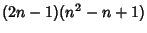Centered Pentagonal Number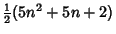Centered Square NumberCentered Triangular Number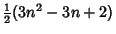Cubic Number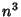Decagonal NumberGnomic NumberHeptagonal NumberHex Number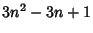Heptagonal Pyramidal NumberHexagonal NumberHexagonal Pyramidal NumberOctagonal Number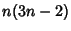Octahedral Number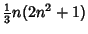Pentagonal NumberPentagonal Pyramidal Number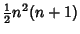Pentatope NumberPronic NumberRhombic Dodecahedral NumberSquare NumberStella Octangula NumberTetrahedral NumberTriangular NumberTruncated Octahedral NumberTruncated Tetrahedral NumberSee also Biquadratic Number, Centered Cube Number, Centered Pentagonal Number, Centered Polygonal Number, Centered Square Number, Centered Triangular Number, Cubic Number, Decagonal Number, Figurate Number Triangle, Gnomic Number, Heptagonal Number, Heptagonal Pyramidal Number, Hex Number, Hex Pyramidal Number, Hexagonal Number, Hexagonal Pyramidal Number, Nexus Number, Octagonal Number, Octahedral Number, Pentagonal Number, Pentagonal Pyramidal Number, Pentatope Number, Polygonal Number, Pronic Number, Pyramidal Number, Rhombic Dodecahedral Number, Square Number, Stella Octangula Number, Tetrahedral Number, Triangular Number, Truncated Octahedral Number, Truncated Tetrahedral Number

References

Conway, J. H. and Guy, R. K. The Book of Numbers. New York: Springer-Verlag, pp. 30-62, 1996.

Dickson, L. E. Polygonal, Pyramidal, and Figurate Numbers.'' Ch. 1 in History of the Theory of Numbers, Vol. 2: Diophantine Analysis. New York: Chelsea, pp. 1-39, 1952.

Goodwin, P. A Polyhedral Sequence of Two.'' Math. Gaz. 69, 191-197, 1985.

Guy, R. K. Figurate Numbers.'' §D3 in Unsolved Problems in Number Theory, 2nd ed. New York: Springer-Verlag, pp. 147-150, 1994.

Kraitchik, M. Figurate Numbers.'' §3.4 in Mathematical Recreations. New York: W. W. Norton, pp. 66-69, 1942.